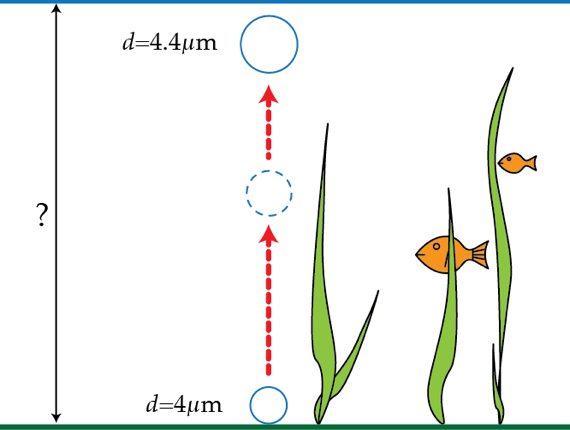# Rising bubble

A small spherical gas bubble of diameter $d=4~\mu \mbox{m}$ forms at the bottom of a pond. When the bubble rises to the surface its diameter is $n=1.1$ times bigger. What is the depth in meters of the pond?Details and assumptions

The atmospheric pressure is $p_{A}=10^{5}~\mbox{Pa}$, the acceleration of gravity is $g=9.8~\text{m}/{\text{s}^{2}}$ and water's surface tension and density are $\sigma=73\times 10^{-3}~\text{N}/\text{m}$ and $\rho=1\times 10^{3}~\text{kg}/\text{m}^3$, respectively. The gas expansion is assumed to be isothermal.

×

Problem Loading...

Note Loading...

Set Loading...